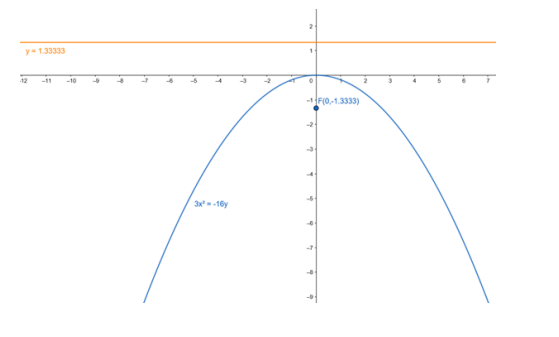# Find the coordinates of the focus and the vertex, the equations of the`
Question:

Find the coordinates of the focus and the vertex, the equations of the directrix and the axis, and length of the latus rectum of the parabola :

$3 x^{2}=-16 y$

Solution:

Given equation :

$3 x^{2}=-16 y$

$x^{2}=-\frac{16}{3} y$

Comparing the given equation with parabola having an equation,

$x^{2}=4 a y$

$4 a=\frac{16}{3}$

$a=\frac{4}{3}$

Focus: $F(0,-a)=F\left(0,-\frac{4}{3}\right)$

Vertex: $A(0,0)=A(0,0)$

Equation of the directrix: $y-a=0$

$y-\frac{4}{3}=0$

$y=\frac{4}{3}$

Lenth of latusrectum :

$4 a=\frac{16}{3}$